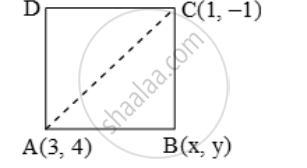# If the Opposite Vertices of a Square Are (1, – 1) and (3, 4), Find the Coordinates of the Remaining Angular Points. - Mathematics

Sum

If the opposite vertices of a square are (1, – 1) and (3, 4), find the coordinates of the remaining angular points.

#### Solution

Let A(1, – 1) and C(3, 4) be the two opposite vertices of a square ABCD and let B(x, y) be the third vertex.Then, AB = BC

⇒ AB^2 = BC^2

⇒ (x – 1)^2 + (y + 1)^2 = (3 – x)^2 + (4 – y)^2

⇒ x^2 – 2x + 1 + y^2 + 2y + 1 = 9 – 6x + x^2 + 16 – 8y + y2

⇒ x^2 + y^2 – 2x + 2y + 2 = x^2 + y^2 – 6x – 8y + 25

⇒ 4x + 10y = 23

⇒ x = \frac { 23-10y }{ 4 } ….(1)

In right-angled triangle ABC, we have

AB^2 + BC^2 = AC^2

⇒ (x – 3)^2 + (y – 4)^2 + (x – 1)^2 + (y + 1)^2 = (3 –1)^2 + (4 + 1)^2

⇒ x^2 + y^2 – 4x – 3y – 1 = 0 …. (2)

Substituting the value of x from (1) and (2),

we get

((23-10y)/4)^2+ y^2 – (23 – 10y) – 3y – 1 = 0

⇒ 4y^2 – 12y + 5 = 0 ⇒ (2y – 1) (2y – 5) = 0

⇒ y = \frac { 1 }{ 2 } or \frac { 5 }{ 2 }

Putting y = \frac { 1 }{ 2 }and y = \frac { 5 }{ 2 } respectively in (1) we get

x = \frac { 9 }{ 2 } and x = \frac { -1 }{ 2 }" respectively"

Hence, the required vertices of the square are ( \frac{9}{2},\frac{1}{2})\text{ and }( -\frac{1}{2},\ \frac{5}{2})

Concept: Distance Formula
Is there an error in this question or solution?